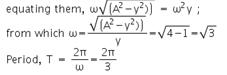Courses

# Test: Simple Harmonic Motion

## 10 Questions MCQ Test Physics Class 11 | Test: Simple Harmonic Motion

Description
This mock test of Test: Simple Harmonic Motion for Class 11 helps you for every Class 11 entrance exam. This contains 10 Multiple Choice Questions for Class 11 Test: Simple Harmonic Motion (mcq) to study with solutions a complete question bank. The solved questions answers in this Test: Simple Harmonic Motion quiz give you a good mix of easy questions and tough questions. Class 11 students definitely take this Test: Simple Harmonic Motion exercise for a better result in the exam. You can find other Test: Simple Harmonic Motion extra questions, long questions & short questions for Class 11 on EduRev as well by searching above.
QUESTION: 1

### The velocity of a particle moving with simple harmonic motion is____ at the mean position.

Solution:

V = ω√(A2 – x2)
So the velocity is maximum at mean position

QUESTION: 2

### The periodic time (tp) is given by

Solution:

Periodic time is the time taken for one complete revolution of the particle.
∴ Periodic time, tp = 2 π/ω seconds.

QUESTION: 3

### A frequency of 1Hz corresponds to:

Solution:

Frequency is defined as time taken to perform one oscillation by the object, hence 1Hz corresponds to 1 vibration per sec.

QUESTION: 4

A second pendulum is mounted in a space shuttle. Its period of oscillations will decrease when rocket is

Solution:

Time Period, T = 2π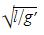; where l = Length of seconds pendulum

g’= Apparent Gravity

=> For the period of oscillations of Seconds Pendulum to decrease, the Apparent gravity (g’) has to increase because-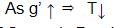Hence, Time Period of oscillations of Seconds Pendulum will decrease when the rocket is ascending up with uniform acceleration.

QUESTION: 5

A particle of mass 10 gm lies in a potential field v = 50 x2 + 100. The value of frequency of oscillations in Hz is

Solution: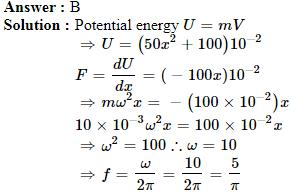QUESTION: 6

If a simple pendulum oscillates with an amplitude 50 mm and time period 2s, then its maximum velocity is

Solution:

We know that in a simple harmonic motion the maximum velocity, vmax = A⍵
Here A = 50 mm
And ⍵ = 2π / T
= 2π / 2
= π
Hence  vmax   = 50 x 10-3
= 0.15 m/s

QUESTION: 7

In simple harmonic motion the displacement of a particle from its equilibrium position is given by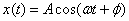. Here the phase of motion is

Solution:
QUESTION: 8

Find the amplitude of the S.H.M whose displacement y in cm is given by equation y= 3sin 157t +4cos157t where t is time in seconds.

Solution:

When the displacement of a SHM is y=a sin wt+ b cos wt, the amplitude of the SHM
will be A=√a2+b2
Here, a=3, b=4 so amplitude A=√32+42=5 cm
Hence option B is correct.

QUESTION: 9

What will be the phase difference between bigger pendulum (with time period 5T/4 )and smaller pendulum (with time period T) after one oscillation of bigger pendulum?

Solution:

After one oscillation of a bigger pendulum i.e. 5T/4, ¼ of the total phase is travelled by the smaller pendulum while the bigger is still at initial position. Thus the phase difference between two is ¼ (2π) - 0 = π/2

QUESTION: 10

A particle executes linear simple harmonic motion with an amplitude of 2 cm. When the particle is at 1 cm from the mean position, the magnitude of its velocity is equal to that of its acceleration. Then its time period in seconds is:

Solution:

The magnitudes of the velocity and acceleration of the particle when its displacement is 'y' are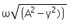and ω2y respectively.